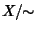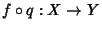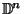## Quotient Space

The quotient spaceof a Topological Spaceand an Equivalence Relation ~ onis the set of Equivalence Classes of points in(under the Equivalence Relation ) together with the following topology given to subsets of: a subsetofis called open Iffis open in.

This can be stated in terms of Maps as follows: ifdenotes the Map that sends each point to its Equivalence Class in, the topology oncan be specified by prescribing that a subset ofis open Iffis open.

In general, quotient spaces are not well behaved, and little is known about them. However, it is known that any compact metrizable space is a quotient of the Cantor Set, any compact connected-dimensional Manifold foris a quotient of any other, and a function out of a quotient spaceis continuous Iff the functionis continuous.

Letbe the closed-D Disk andits boundary, the-D sphere. Then(which is homeomorphic to), provides an example of a quotient space. Here,is interpreted as the space obtained when the boundary of the-Disk is collapsed to a point, and is formally the quotient space by the equivalence relation generated by the relations that all points inare equivalent.''